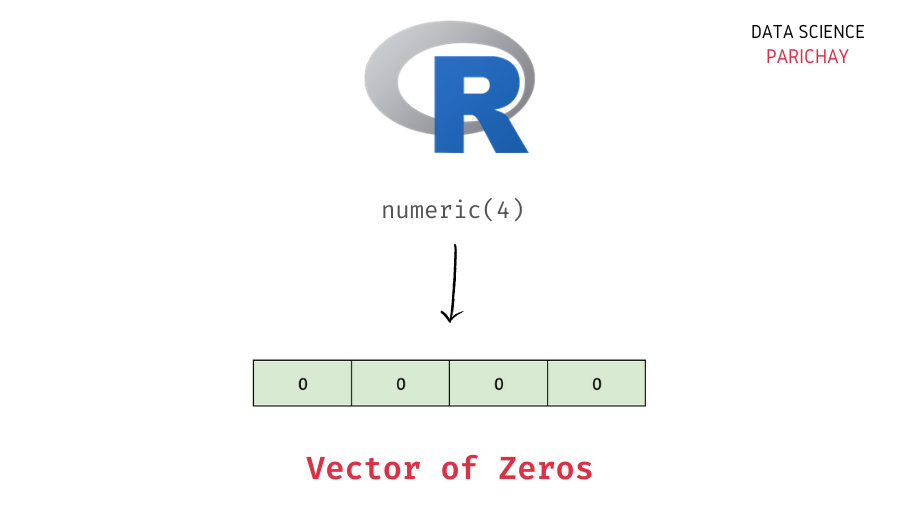# Create a Vector of Zeros in R

In this tutorial, we will look at how to create a vector of zeros (having 0 as its elements) in R with the help of some examples.

## How to create a vector of zeros in R?

There are multiple ways to create a vector for zeros. Some of the functions that can help you create a vector of zeros in R are – `c()`, `integer()`, `numeric()`, `rep()`, etc.

Let’s look at these methods with the help of examples.

### Using the `c()` function

The `c()` function is used to combine different values (and/or vectors) into a vector. If you want to create a vector of zeros with a relatively smaller length, you can use the `c()` function.

For example, let’s use the `c()` function to create a vector of four zeros.

```# create a vector of zeros
vec <- c(0, 0, 0, 0)
# display the vector
print(vec)```

Output:

` 0 0 0 0`

We get a vector of four zeros. Note that, manually typing zeros can become cumbersome when you want to create a vector of zeros of a larger length. In such cases, you can use the methods described below.

### Using the `integer()`function

Pass the length of the vector as an argument to the `integer()` function. For example, let’s create a vector of four zeros.

📚 Data Science Programs By Skill Level

Introductory

Intermediate ⭐⭐⭐

🔎 Find Data Science Programs 👨‍💻 111,889 already enrolled

Disclaimer: Data Science Parichay is reader supported. When you purchase a course through a link on this site, we may earn a small commission at no additional cost to you. Earned commissions help support this website and its team of writers.

```# create a vector of zeros
vec <- integer(4)
# display the vector
print(vec)```

Output:

` 0 0 0 0`

You can see that we pass 4 as an argument to the `integer()` function. We get a vector of four zeros. Note that, here, the resulting data type of values is `integer` and not `numeric` (that we get in the other methods).

### Using the `numeric()` function

You can similarly use the `numeric()` function to create a vector of zeros. It also takes the length of the vector you want to create as an argument. Let’s look at an example.

```# create a vector of zeros
vec <- numeric(4)
# display the vector
print(vec)```

Output:

` 0 0 0 0`

We get the same result as above.

### Using the `rep()` function

The `rep()` function is used to replicate a value a specified number of times.

To create a vector of zeros using the `rep()` function, pass `0` as the first argument and the vector length as the second argument. For example, let’s use the `rep()` function to create a vector of four zeros.

```# create a vector of zeros
vec <- rep(0, 4)
# display the vector
print(vec)```

Output:

` 0 0 0 0`

We get the same result as above.

You might also be interested in –

•